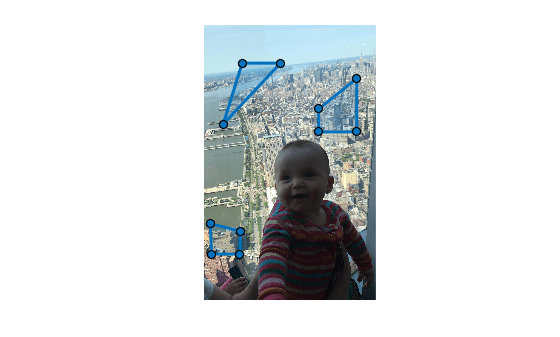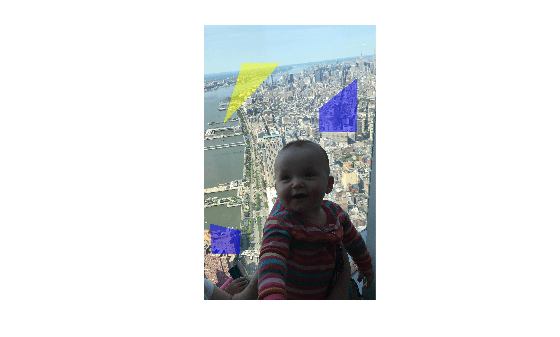poly2label

Create label matrix from set of ROIs

Description

example

L = poly2label(ROIpositions,ROILabelIDs,imagesize) creates a numeric label matrix L from the regions of interest (ROIs) defined in ROIpositions. ROILabelIDs specifies the numeric ID for each ROI in ROIpositions. imagesize specifies the size of the output label matrix.

L = poly2label(ROIpositions,ROILabelIDs,R) creates a numeric label matrix where the spatial referencing object R specifies the coordinate system used by the ROI positions in ROIpositions. The function assumes that the ROI positions are in world limits defined by R. The ImageSize property of R specifies the size of the label matrix L.

Examples

collapse all

Read an image into the workspace and display it.

figure
imshow(I)

Initialize the ROI position cell array and image size variables. If you pass poly2label a size value containing three dimensions, it only uses the first two, m-by-n.

numPolygon = 3;
roiPositions = cell(numPolygon,1);
imSize = size(I);

Specify the coordinates of three ROIs in the roiPositions cell array. In this example, the first ROI is a triangle, requiring coordinates for three corners. The other two ROIs are quadrilaterals, requiring coordinates for four corners.

roiPositions{1} = [500 500; 250 1300; 1000 500];
roiPositions{2} = [1500 1100; 1500 1400; 2000 1400; 2000 700];
roiPositions{3} = [80 2600; 480 2700; 470 3000; 100 3000];

Create an array for label IDs the same size as the roiPositions cell array.

roilabelID = zeros(numPolygon,1,'uint8');

Specify label ID values that correspond to the order in which you listed the ROIs in roiPositions. The first ROI is a triangle so give it the label 1. The next two ROIs are both quadrilaterals so give them the label 2.

roilabelID(1) = 1;
roilabelID(2) = 2;
roilabelID(3) = 2;

Draw the three ROIs on the figure.

for id = 1:numPolygon
drawpolygon('Position',roiPositions{id});
endCreate a label matrix from the ROIs. The label matrix is the same size, m-by-n, as the original image.

L = poly2label(roiPositions,roilabelID,imSize);

Display the label matrix overlaid on the original image.

figure;
B = labeloverlay(I,L);
imshow(B);Input Arguments

collapse all

Coordinate vectors, specified as a 1-by-P cell array of numeric coordinate vectors, where P is the total number of ROIs. Each cell array element is an s-by-2 coordinate vector of the form [x1 y1; …; xs ys], where s is the total number of vertices for that ROI. Each x,y pair defines a vertex of the ROI. If the ROI shape is not already closed, the poly2label function closes the shape automatically. You can specify any number of ROIs.

Data Types: double | cell

Labels for each ROI, specified as a numeric vector of the same length as the ROIpositions argument. Each label in the vector corresponds to the ROI in the associated position in the ROIpositions cell array.

poly2label assigns the value 0 to all background pixels in the output image.

Data Types: single | double | int8 | int16 | int32 | uint8 | uint16 | uint32

Size of the output label matrix, specified as a 2- or 3-element numeric vector. If you specify a 3-element vector, the poly2label function uses only the first two dimensions, m-by-n.

Data Types: double

Spatial referencing information, specified as an imref2d object.

Output Arguments

collapse all

Label matrix, returned as an m-by-n matrix of nonnegative values, the same class as ROIlabelIDs. Pixels labeled 0 are the background.

Tips

• The poly2label function sets pixels that are inside an ROI to a label value. For information about how poly2label handles pixels that are only partially inside an ROI, see the poly2mask function.

• When the positions of several ROIs overlap each other, the ROI label with the lowest index number in the ROIpositions cell array overwrites the other ROIs.